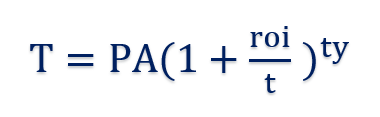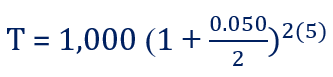# Compound Interest Formula

How to calculate compound interest

## What is the Compound Interest Formula?

To start, it’s important to understand first what compound interest is. Compound interest is taken from the initial – or principal – amount on a loan or a deposit, plus any interest that has already accrued. The compound interest formula is the way that such compound interest is determined.Compound interest accrues over the period a loan or a deposit is outstanding. How it accrues depends on how often it compounds. The compound interest will be higher, the more compounding periods there are. What exactly does that mean? If, for example, a \$1,000 loan comes with a 2% semi-annual compounding interest rate, it will generate a more accrued compound interest than the same loan amount that is compounded at 4% annually.

### Summary:

• Compound interest is based on the amount of the principal of a loan or deposit – and interest rate – which accrues in conjunction with how often the loan compounds: typically, compounding occurs either annually, semi-annually, or quarterly.
• The compound interest formula is the way that compound interest is determined.
• Compound interest is valuable for those who make deposits because it is an additional income for them the longer the deposit sits without withdrawals. It is valuable to lenders because it represents additional income earned on money lent.

### How to Calculate Compound Interest

The compound interest formula is as follows:Where:

• T = Total accrued, including interest
• PA = Principal amount
• roi = The annual rate of interest for the amount borrowed or deposited
• t = The number of times the interest compounds yearly
• y = The number of years the principal amount has been borrowed or deposited

### Practical Example

Let’s put some numbers into the above formula to make it clearer. For this example, let’s say that a \$1,000 loan is offered, with an interest rate of 5%, which is compounded semi-annually. If the loan is extended for five years, what would the balance for repayment be?After five years, the total amount owed would be \$1,280.08. The calculation would work in the same way when speaking of a \$1,000 amount deposited into a bank receiving the same compounded interest. After five years, the total would be valued at \$1,280.08.

It should be noted that in solving only for compound interest, the principal amount of the loan or deposit would need to be subtracted from the total.

Compound interest is of great importance for those who have deposited money or made an investment because it enables them to earn an increasing amount of income off of an initial investment. It is valuable to lenders because it adds additional income on top of the amount lent to a borrower.

CFI offers the Financial Modeling & Valuation Analyst (FMVA)™ certification program for those looking to take their careers to the next level. To keep learning and advancing your career, the following CFI resources will be helpful:

• Annual Percentage Yield
• Effective Annual Interest Rate
• Continuously Compounded Interest
• Interest Income

### Financial Analyst Training

Get world-class financial training with CFI’s online certified financial analyst training program!

Gain the confidence you need to move up the ladder in a high powered corporate finance career path.

Learn financial modeling and valuation in Excel the easy way, with step-by-step training.